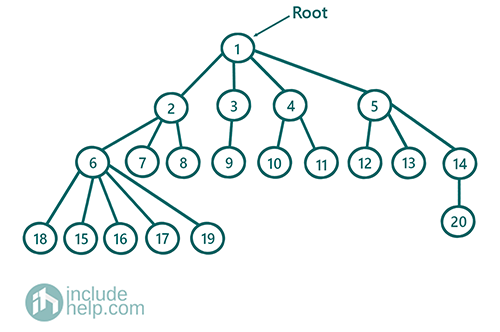# Find the height of an N-array tree

In this article, we are going to see how to find the height of an n-array tree both recursively and iteratively?
Submitted by Radib Kar, on July 31, 2020

Prerequisite:

In the previous tutorial, we saw how to represent an n-array tree & its traversals? There we saw two types of traversals,

1. Depth-First Search Traversal
2. Breadth-First Search Traversal

We can use both the traversal to find the height.In the above n-array tree the height is 4
Level0-> root only 1
Level1-> 2 ,3, 4, 5
Level2-> 6 ,7, 8, 9, 10, 11, 12, 13, 14
Level3-> 15, 16, 17, 18, 19, 20

## 1) Finding height recursively using depth First search traversal

We can use recursive traversal and find the height.

Height of a tree would be 1+maximum height of the children

To find the maximum height we can use the same recursive function like below:

```int height(TreeNode root)
if(root is NULL)
return 0
if(root is a leaf node)
return 1
max_children_height=INT_MIN
for each children c:
max_children_height=max(max_children_height, height(c))
return 1+max_children_height
End Function
```
```#include <bits/stdc++.h>
using namespace std;

class TreeNode {
public:
int val;
vector<TreeNode*> children;
TreeNode(int v)
{
val = v;
}
TreeNode(int v, int n)
{
val = v;
children = vector<TreeNode*>(n, NULL);
}
};

//recursive function
int height(TreeNode* root)
{
if (!root)
return 0;
//leaf node
if (root->children.size() == 0) {
return 1;
}

int max_child_height = INT_MIN;
//height=1+max(subtree heights)
//subtree heights found recursively
for (int i = 0; i < root->children.size(); i++) {
max_child_height = max(max_child_height, height(root->children[i]));
}
return 1 + max_child_height;
}

int main()
{
TreeNode* root = new TreeNode(1, 4);

root->children = new TreeNode(2, 3);
root->children = new TreeNode(3, 1);
root->children = new TreeNode(4, 2);
root->children = new TreeNode(5, 3);

root->children->children = new TreeNode(6, 5);
root->children->children = new TreeNode(7);
root->children->children = new TreeNode(8);

root->children->children = new TreeNode(9);

root->children->children = new TreeNode(10);
root->children->children = new TreeNode(11);

root->children->children = new TreeNode(12);
root->children->children = new TreeNode(13);
root->children->children = new TreeNode(14, 1);

root->children->children->children = new TreeNode(15);
root->children->children->children = new TreeNode(16);
root->children->children->children = new TreeNode(17);
root->children->children->children = new TreeNode(18);
root->children->children->children = new TreeNode(19);

root->children->children->children = new TreeNode(20);
cout << "Height of the tree is: " << height(root);

return 0;
}
```

Output:

```Height of the tree is: 4
```

## 2) Finding height iteratively using breath First search traversal

We can do this using level order traversal as we did in finding the height of a binary tree using level order traversal.

So here also what we have to do is to distinguish b/w two consecutive levels and we will increment a counter at the start of each level. To distinguish b/w levels or to indicate the end of a level, we inject a dummy NULL Node like below algorithm.

```1)  Initialize a queue q
2)  Push root node to the queue and then push NULL to indicate end of level0
3)  Set counter 0
4)  while(queue, q is not empty){
temp=DeQueue(q);  //Dequeue
if(temp==NULL){
indicates the end of the last level reached
So increment counter
//that means there are still some nodes of next level
If(queue, q is not empty())
EnQueue(NULL)
}
Else{
Push all children to the queue q
}
End While
5)  counter will give the result which is the height of the tree
```
```#include <bits/stdc++.h>
using namespace std;

class TreeNode {
public:
int val;
vector<TreeNode*> children;
TreeNode(int v)
{
val = v;
}
TreeNode(int v, int n)
{
val = v;
children = vector<TreeNode*>(n, NULL);
}
};

//recursive function
int height(TreeNode* root)
{

queue<TreeNode*> q;
q.push(root);
q.push(NULL);
int counter = 0;
while (!q.empty()) {
TreeNode* temp = q.front();
q.pop();
//end of last level
if (temp == NULL) {
counter++;
if (!q.empty()) {
//indicate end of the current level
q.push(NULL);
}
}
else {
for (int i = 0; i < temp->children.size(); i++)
q.push(temp->children[i]);
}
}
return counter;
}

int main()
{
TreeNode* root = new TreeNode(1, 4);

root->children = new TreeNode(2, 3);
root->children = new TreeNode(3, 1);
root->children = new TreeNode(4, 2);
root->children = new TreeNode(5, 3);

root->children->children = new TreeNode(6, 5);
root->children->children = new TreeNode(7);
root->children->children = new TreeNode(8);

root->children->children = new TreeNode(9);

root->children->children = new TreeNode(10);
root->children->children = new TreeNode(11);

root->children->children = new TreeNode(12);
root->children->children = new TreeNode(13);
root->children->children = new TreeNode(14, 1);

root->children->children->children = new TreeNode(15);
root->children->children->children = new TreeNode(16);
root->children->children->children = new TreeNode(17);
root->children->children->children = new TreeNode(18);
root->children->children->children = new TreeNode(19);

root->children->children->children = new TreeNode(20);
cout << "Height of the tree is: " << height(root);

return 0;
}
```

Output:

```Height of the tree is: 4
```

What's New (MCQs)

Top Interview Coding Problems/Challenges!

Languages: » C » C++ » C++ STL » Java » Data Structure » C#.Net » Android » Kotlin » SQL
Web Technologies: » PHP » Python » JavaScript » CSS » Ajax » Node.js » Web programming/HTML
Solved programs: » C » C++ » DS » Java » C#
Aptitude que. & ans.: » C » C++ » Java » DBMS
Interview que. & ans.: » C » Embedded C » Java » SEO » HR
CS Subjects: » CS Basics » O.S. » Networks » DBMS » Embedded Systems » Cloud Computing
» Machine learning » CS Organizations » Linux » DOS
More: » Articles » Puzzles » News/Updates

© https://www.includehelp.com some rights reserved.